< Prev
Next >

# C - Primitive Data Types and Sizes

In C, each variable has a specific data type, where a data type tells us the size, range and the type of a value that can be stored in a variable. In C, there are about seven primitive data types. These data types are : short, int, long, char, float, double and few of their variants.

## Primitive data types are categorized into these parts

• integer data types, such as short, int, long.
• floating-point data types, such as float, double.
• character data type, such as char.

## Primitive Data types in C

In C, the number of bytes used to store a data type depends on the Compiler(depending on the bit size of a compiler and also the OS). But irrespective of the bit-size of the compiler and OS, the following rules are followed, such as -

Data Type Size Range
char at least 1 byte -128 to 127
unsigned char at least 1 byte 0 to 255
short at least 2 bytes -32768 to 32767
unsigned short at least 2 bytes 0 to 65535
int at least 2 bytes -32768 to 32767
unsigned int at least 2 bytes 0 to 65535
long at least 4 bytes -2,147,483,648 to 2,147,483,647
unsigned long at least 4 bytes 0 to 4,294,967,295
float at least 2 bytes 3.4e-038 to 3.4e+038
double at least 8 bytes 1.7e-308 to 1.7e+308
long double at least 10 bytes 1.7e-4932 to 1.7e+4932

## How to calculate range of a data type?

For many of us who like the reasoning behind equations. We will use a formula to calculate a minimum range and a maximum range for the data types like short, char, int and long.

Minimum Range- It is the minimum value that can be stored in a data type.
Maximum Range- It is the maximum value that can be stored in a data type.

The minimum range is calculated by -2(bits-1) and for the maximum range it is 2(bits-1)-1, while bits = size of a data type.

For example, A short variable of size takes at least 2 bytes(16 bits) in size. And, as per formula above. The minimum range is -215(-32768) and the maximum range is 215-1(32767). Hence, the range of values for short data type is -32768 to 32767.

## short

C provides a variant of an integer data type called short. Short integer was designed to provide integer values of smaller range than an int data type.• In C, the number of bytes used to store a data type depends on the Compiler(depending on the bit size of a compiler and also the OS), although the size of a short variable is at least 16 bits or 2 bytes and never bigger than size of int.

Hence, for a short variable of 2 bytes, the minimum value that can be stored in a short is -215 or -32768 and a maximum is 215-1 or +32767. So, it's range is -32768 to 0 to 32767.

## unsigned short

When we already know that we only wish to store a positive short integer value then we could use a variant of an short data type called unsigned short. The unsigned short data type is used to store only positive short integer values.It stores double more positive short integer values than an short data type, because the highest bit which is usually occupied to store the sign of an integer value is now free and not used to store the sign of the integer.

Hence, for an unsigned short variable of 2 bytes, the minimum value that can be stored is 0 and a maximum is 216 or 65535. So, it's range is 0 to 0 to 65535

## int

In C, the number of bytes used to store a data type depends on the Compiler. Depending on the bit size of a compiler and also the OS, although the size of an int variable is at least 16 bits or 2 bytes and never bigger than long.

For example -

• A 16-bit Turbo C/Turbo C++ Compiler takes at least 2 bytes to store an int, where the
minimum value that can be stored in an int is -215 or -32768 and the maximum value is 215-1 or 32767.

•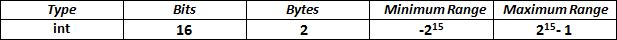• Where as, a 32-bit compiler like Visual C++ or GCC would reserve 4 bytes to store an int, where the minimum value that can be stored in an int is -231 or -2, 147, 483, 648 and the maximum value is 231-1 or 2, 147, 483, 647.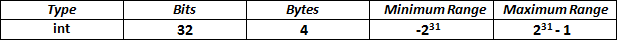Compiler decides an appropriate size of a data type depending on the operating system and hardware for which it is written.

The highest bit decides the sign of an integer i.e. if the highest bit is 1 then the integer value is negative and if the highest bit is 0 then the integer value is positive. The int data type could be signed and unsigned.

By default an int data type stores both positive and negative integer values, hence it could also be termed as a signed int data type, which means it stores both positive and negative integer values.

## unsigned int

When we already know that we wish to store a positive integer value then we could use a variant of an int data type called unsigned int. The unsigned int data type is used to store only positive integer values.It stores double more positive integer values than an int data type, because the highest bit which is usually occupied to store the sign of an integer value is now free and not used to store the sign of the integer.

• Depending on the bit size of a compiler and also the OS, for a unsigned int variable of 2 bytes, the minimum value that can be stored is 0 and a maximum is 216 or 65535. So, it's range is to 0 to 65535.

• Depending on the bit size of a compiler and also the OS, for a unsigned int variable of 4 bytes, the minimum value that can be stored is 0 and a maximum is 232 or 4,294,967,295. So, it's range is to 0 to 4,294,967,295.

## long

• Depending on the bit size of a compiler and also the OS, the size of a long variable in C is at least 32 bits or 4 bytes.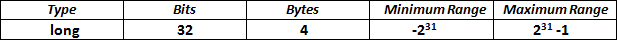The minimum range of a value that can be stored in a long is -231 while, the maximum is 231-1
Note : Because of a wider range than int, long data type is used to store a larger number.

• ## unsigned long

When we already know that we wish to store a positive long integer value then we could use a variant of an long data type called unsigned long. The unsigned long data type is used to store only positive long integer values.It stores double more positive integer values than an long data type, because the highest bit which is usually occupied to store the sign of an integer value is now free and not used to store the sign of the integer.

• For a unsigned int variable of 4 bytes, the minimum value that can be stored is 0 and a maximum is 232-1 or 4,294,967,295. So, it's range is to 0 to 4,294,967,295.

• ## float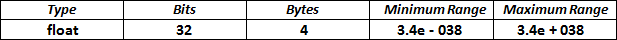• In float data types, we can hold number with its fractional part as well. So, we use this data type when a very precise calculation is required. Size of a float variable is at least 32 bits = 4 bytes.

• You don't need to remember the minimum and maximum value floating data types but for those who still wish to know, its minimum range is 3.4e-038 and a maximum limit of 3.4e+038.

• Note : By default, any floating-point value defaults to data-type double, hence an f suffix is used to denote a literal of type float.

• ## double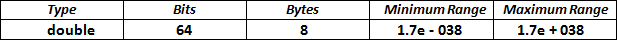• Size of a double variable is at least 64 bits= 8 bytes. Double data type variable may store a data with a minimum value of 1.7 e-038 until a maximum value of 1.7e+038
Note : In double data type, we can hold numbers with double precision values, as compared to float.

• ## char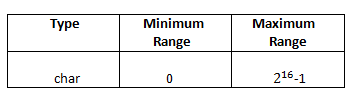• C provides a char data type used to store character values. The char data type could be signed and unsigned.

You don’t have to specify the keyword signed when declaring a signed char variable, but you need to specify the keyword unsigned for declaring a unsigned char variable.

Now you would think how a character can be signed(positive or negative signed)?

Well, when you store a character value using char data type, what gets stored is not the character but the binary equivalent of ASCII value of the character.

For example :
``char c= 'A';``

This code stores the binary equivalent of ASCII value of A i.e. 65 in the system. And if 65 could be stored using a char data type then even a negative integer value could be stored using a char data type. It usually takes one byte to store a character using char data type. In C char type variable usually takes 8 bits= 1 byte in the memory. Hence, for a char variable of 1 byte, minimum range is 28-1 and the maximum range is 28-1. Ranging from -128 to 127 values.

• ## Note :

C does not provide a data type for representing boolean values to represent boolean values.

## Format specifiers in console formatted I/O functions

Some of the most commonly used format specifiers in input/output functions to display data types in C language are displayed in the table below -

Format Specifiers Description
%hi

Format specifier used to read or display a signed short integer value or short integer value.

%hu

Format specifier used to read or display an unsigned short integer value.

%d

Format specifier used to read or display a signed int integer value.

%u

Format specifier used to read or display a unsigned int integer value.

%ld

Format specifier used to read or display a long integer value or signed long integer value.

%lu

Format specifier used to read or display a unsigned long integer value.

%c

Format specifier used to read or display a char, character value. Format specifier %hhi is used to display a signed numerical value.

%c

Format specifier used to read or display a unsigned char, character value. Format specifier %hhu is used to display a signed numerical value.

%f

Format specifier used to read or display a float, floating-point value.

%lf

Format specifier used to read or display a double, floating-point value.

%Lf

Format specifier used to read or display a long double, floating-point value.

%s

Format specifier used to read or display a string value, to be stored in a char[] array.

## Example with all primitive data types

Note : We are going to use a line break by using a newline character i.e. "\n" in our program, which takes the cursor to the next line.

``````#includ<stdio.h>

int main()
{
char ch1 = -128;
char ch2 = 'a';
unsigned char uc='b';
short s = 10;
int i = 1000;
unsigned int ui = 45555;
long l = 1234567;
unsigned long ul = 1234567898;
float f = 3.5f;
double d = 23.9999;
long double ld = 23.239;

printf("char value : %c \n", ch1);
printf("signed numerical value of char : %hhi \n", ch1);
printf("char value : %c \n", ch2);
printf("unsigned char value : %c \n", uc);
printf("unsigned numerical value of char : %hhu \n", uc);
printf("short value : %hi \n", s);
printf("int value : %d \n",i);
printf("unsigned int value : %u \n", ui);
printf("long value : %ld \n", l);
printf("unsigned long value : %ul \n", ul);
printf("float value : %f \n ", f);
printf("double value : %lf \n", d);

return 0;
}``````

## Output

``````char value : Ç
signed numerical value of char : -128
char value : a
unsigned char value : b
unsigned numerical value of char : 98
short value : 10
int value : 1000
unsigned int value : 45555
long value : 1234567
unsigned long value : 1234567898l
float value : 3.500000
double value : 23.999900``````

< Prev
Next >
< The First C Program
Variables, Keywords, Constants >